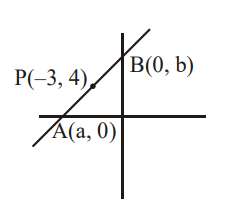# If a straight line passing thourgh the point`
Question:

If a straight line passing thourgh the point $P(-3,4)$ is such that its intercepted portion between the coordinate axes is bisected at $\mathrm{P}$, then its equation is:

1. $x-y+7=0$

2. $3 x-4 y+25=0$

3. $4 x+3 y=0$

4. $4 x-3 y+24=0$

Correct Option: , 4

Solution:Let the line be $\frac{x}{a}+\frac{y}{b}=1$

$(-3,4)=\left(\frac{\mathrm{a}}{2}, \frac{\mathrm{b}}{2}\right)$

$a=-6, b=8$

equation of line is $4 x-3 y+24=0$